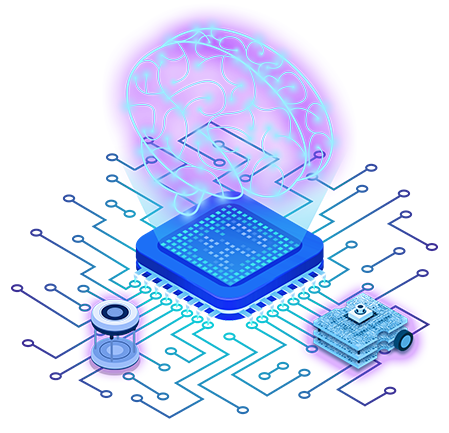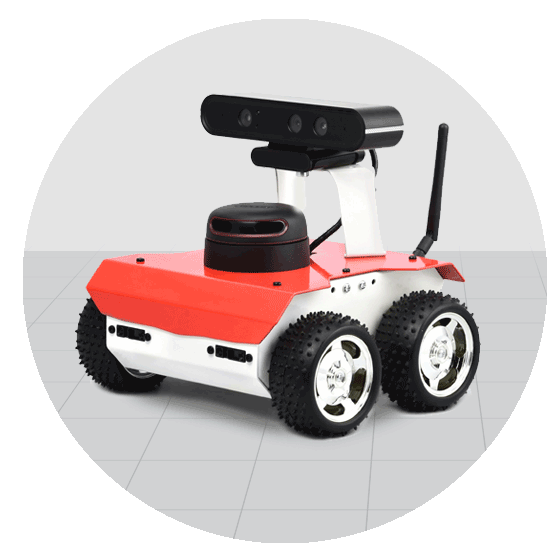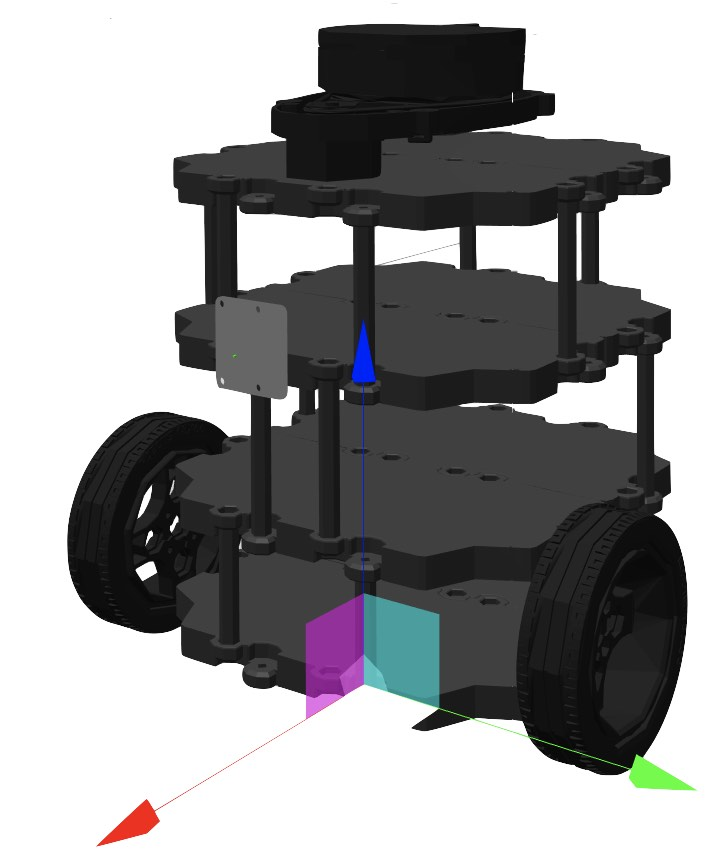# Deep Learning for Robotics Course - Python

This course is about learning the basics of Deep Learning using the super cool Neural Networks!## Course Summary

Deep Learning is a powerful technique to address problems that were previously unbearable for society, due to the difficulty of translating them into computers, their computational cost, or their mathematical complexity.

With good supervision and critical thinking, many new algorithms can be designed using Neural Networks, but you are going to need to put into practice your previous knowledge of mathematics, statistics, and computer programming.

## What you will learn

In this course, we will assume a previous background in mathematics and statistics applications. Their concepts will be used within Python code, classes and functions, and useful libraries such as Keras and Tensorflow.

Also, we will assume previous knowledge of some basic ROS functionalities, on how to create packages, start simulations, run ROS nodes, and compile their code.

You'll be presented with the two typical challenges for unsupervised learning: regression and classification algorithms. You are going to see the key components of a neural network, neurons, and understand the role of weights, biases, activation functions, loss, and accuracy.

You'll be presented with the hyperparameters that rule the behavior of a neural network. You'll learn how by inspecting the learning evolution of our algorithm we can choose to apply one or another optimization technique to improve its results.

You'll be presented with an example of a Convolutional Neural Network, an excellent tool to solve Computer Vision problems. You'll see which neurons and mathematical operations allow extracting features of images, and then classify them into labels. You are going to be challenged to improve their performance of them with optimization techniques.

## Course Overview

### Introduction to the Course

A brief introduction to the course contents.

### Deep Learning Basics

In this unit you are going to learn how to address a data problem using a Neural Network. You will see and understand the basic mathematics behind them, and what structure should a NN follow. Moreover, you will experiment with Regression and Classification examples from a robotics point of view, using data from our ROS simulator. You will perform these exercises in Python and the library Keras from Tensorflow.

### How to program an L-layer Neural Network in Python

In this unit we are going to set the theory apart, and you are going to learn how to program a whole Neural Network from scratch. It will be very important here to remember your knowledge of mathematics and Python programming. Here, we will be guiding you through all the necessary steps of building a Neural Network with any extra library, and we'll be presenting again the formulas of the previous unit when they are necessary.

### Hyperparameter tuning

In this chapter we are going to review the hyperparameters of the basic structure of a Neural Network. Also, we are going to explain how to organize data to input a NN, and use its output error to get information about its performance. Finally, we are going to present some useful techniques to increase the performance and prediction of our model, and see what parameters we'll need to remember this time.

### Convolutional Neural Networks

In this chapter we are going to review a special example of networks, called Convolutional Neural Network, or CNN. Their popularity never stops growing, and we will present them as a powerful tool to solve Computer Vision problems.We will present the mathematics of these nets, and how they make use of traditional Computer Vision techniques within their neurons and connections.As we did with basic NN, we will be talking about neurons, connections, weights, biases, hyperparameters, and design, training and prediction phases.

### Final project

Build a Computer Vision system integrated with ROS. You will use the ROS world you have in the Real Robot Lab, which contains people, line roads, and traffic signs in simulation.Your main goal in this project will be to build a Convolutional Neural Network that can recognize pictures of traffic signs and classify them into '30 km/h speed limit', '50km/h speed limit', 'Stop', 'Pedestrians only', etc.

## Teachers

### Irene Pérez

My name is Irene, I'm a Physicist and Engineer from Barcelona. I'm passionate about applying AI to Robotics, while focusing in the utility and beauty of fundamental mathematics and physics involved. ROS gives me the tools to enjoy all of that!## Robots used

### ROSbot 2.0 robot### TurtleBot 3 robot## Learning Path

Machine Learning for Robots